Click Question to Get Free Answers >

# Introduction To Euclids Geometry NCERT Solutions : Class 9 Maths

## NCERT Solutions for Class 9 Maths : Introduction To Euclids GeometryFilter
Filters :
Classes
•
•
•
•
•
•
•
Chapters
•
•
•
•
•
•
•
•
•
•
• 7 More
Exercises
•
•
•

### NCERT Class 9 | INTRODUCTION TO EUCLIDS GEOMETRY | Solved Examples | Question No. 03

Consider the following statement: There exists a pair of straight lines that are everywhere equidistant from one another. Is this statement a direct consequence of Euclid's fifth postulate? Explain.Doubtnut one of the best online education platform provides free NCERT Solutions of Maths for Class 9 Introduction To Euclids Geometry which are solved by our maths experts as per the NCERT (CBSE) guidelines. We provide the solutions as video solutions in which concepts are also explained along with answer which will help students while learning , preparing for exams and in doing homework. These Solutions will help to revise complete syllabus and score more marks in examinations.

Get here free, accurate and comprehensive NCERT Solutions for Class 9 Maths Introduction To Euclids Geometry which have been reviewed by our maths counsellors as per the latest edition following up the CBSE guidelines. We provide video solutions in which solutions to all the questions of NCERT Class 9 Maths textbook are explained in step by step and detailed way.

## The Topics of the Chapter Introduction To Euclids Geometry are :

INTRODUCTION

PARALLEL LINES AND INTERSECTING LINES

LINE SEGMENT

It contains these exercises along with solved examples. All the exercises are solved in the video. Select the exercise to view the solutions exercisewise of the Chapter Introduction To Euclids Geometry:

## NCERT Solutions Class 9 Maths Chapter Introduction To Euclids Geometry Exercises:

We have covered all the exercises and also Solved examples in the videos. Along with the practise exercise Students should also practise solved examples to clear the concepts of the Introduction To Euclids Geometry. If incase you have any doubt you can watch the solutions for the given questions.Watch the solutions for the given questions in which it is also explained in the video steps to solve the questions along with answers.

# NCERT Book Class 9 Solutions Chapter 5 Introduction to Euclid’s Geometry

Mathematics is a very important subject that helps us several aspects in our day to day lives. The pattern of teaching mathematics has evolved over the years, from focusing on the theoretical approach to implementing a practical fun-based approach. The pattern of teaching mathematics has transformed so that the students can understand each and every concept in detail and with perfection. The NCERT book plays a vital role in educating the students with the best mathematics knowledge and helps in improving the student’s mathematics skills. In India, for all the competitive examination, the first preference of CBSE board students is the NCERT book, as it provides the students with accurate knowledge and covers entire syllabus as per CBSE board, which helps the students in excelling in their academic exams and also, to successfully compete in the competitive exams for admissions in prestigious academic institutions like IIT-JEE, NIT and many others.

The NCERT book offers the students with a systematic presentation of concepts and methods. The books provide students with numerous examples, which helps in better practice. In addition to this, the unsolved questions help the students to practice more. With all these different practice opportunities, the students will be able to achieve bigger mathematics goals, which will help in developing a strong and successful future.

In all the competitive examination, NCERT books play a vital role. The language in the NCERT math book is simple and easy that explains all the formulas in depth, offers various tips, tricks, and hints that can be utilized in various ways for solving different mathematical questions. For the students of class 9, the NCERT book will be a stepping-stone, to build strong mathematics base, which will help in excelling in the board exam. For the students, the NCERT book provides with the opportunity of hard work and timely revision. At the end of each chapter in the NCERT math book, the complete chapter has been summarized, so the students can revise the complete chapter in a quick and easy way.

Students should make proper notes while studying from the NCERT math book. These notes should be revised from time to time and the students should practice them, for solving the unsolved questions of the book. This repetitive revision and efforts will help the students in strengthening their knowledge and will help them for a longer time period in a fruitful way.

In addition, Doubtnut, an online education platform, makes it easier for students to understand the concepts and theories of chapters. Our online portal provides video-tutorials and thoroughly explains each and every concept in a fun way that is easily understood and remembered by students. Our website also provides a pdf format of the NCERT book Class 9 so that students can crosscheck the methodologies used in the book with our platform without even looking for their book. They can do so just by a click on their laptop or can also download the app for easy access to the learning material anytime of the day.

## Introduction to Euclid’s Geometry Class 9 Solutions

Chapter 5 in the NCERT book Class 9 for Math is Introduction to Euclid’s Geometry. The NCERT solutions for class 9 maths include several exercises on the chapter of Euclid’s Geometry to help students practice and learn the concepts of the geometry more effectively. The solutions given in the book are detailed with easy explanations so that the students can easily comprehend and understand the concepts in detail. Solutions for the chapter are in the form of explanations; solved exercises, as well as problems for practice, are also mentioned. Not only these solutions are a great help to get better results in exams but also help in completing homework and understanding it better.

### Introduction to Euclid’s Geometry

Euclid was a teacher of mathematics at Alexandria in Egypt, and he was popularly known as ‘Father of Geometry”. He introduced the method of proving mathematical results by using deductive logical reasoning (means before any conclusion all the possibilities should be checked if they are true then on the basis of that resultant logic is followed).

### Definitions of Euclid’s

Euclid told that the geometry is an abstract model of the world, which we can see around us. Like that of line, plane, surface, etc.

We can define it as follows: -

1. Any dot that has no surface is called as Point.

2. A mark made by pen or pencil can be straight, and in standing position, means vertical and horizontal that can be called as Line.

3. The line with two specific ends is called a line segment.

5. Any object with length, breadth, and height is called Surface.

6. The edges of a surface are lines.

The students are benefited by these solutions as it helps them understand the concept of the chapter in a better and easy way. Euclid’s system is considered as an extremely deductive, comprehensive and logical approach towards geometry.

It is about shapes, lines, and angles, the students need to have an in-depth understanding of the topics to be able to understand how the shapes, lines, and angles interact with each other.

## Line segment

The figure below is showing line l which has two specific points A and B, which connect each other, therefore the connected part is called (segment), i.e. a line with endpoints at A and B is called the line segment AB.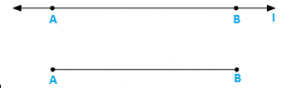### Collinear point

If there are three or more points in the same single line than those points are called collinear points. As shown in the figure below: P, Q, and R are called collinear points.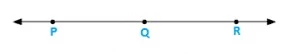### Parallel lines

When two lines do not intersect each other at any point and are in the opposite direction of each other then they are said to be as parallel lines. Line l and m are examples of parallel lines.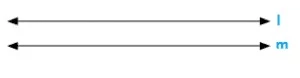### Intersecting lines

When two lines intersect each other at a point, which is common to both lines. Then the lines are called intersecting lines and the point is called the point of intersection.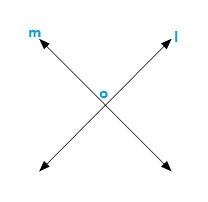### Concurrent lines

When three or more lines are intersecting each other with a common point, these lines are said to be concurrent lines.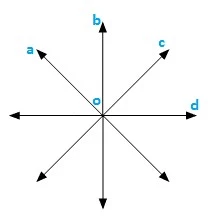### Ray

A line which has one fixed end-point and can be extended from another point infinitely is called ray. In the figure below ‘O’ is a fixed end-point of the  line and point a can be extended till infinity.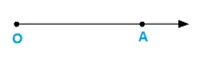### Half-line

If A, B, and C be the points on a line l, such that A lies between B and C, and we delete the point A from line l, the two parts of l that remain are each   called half-line.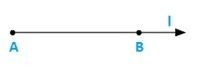Solutions:

Question: 1

(i) How many lines can be drawn through a given point?

(ii) In how many points can two distinct lines at the most intersect?

Solution:

(i) Infinitely many

(ii) One

Question: 2

(i) Given two points P and Q. Find how many line segments do they determine.

(ii) Name the line segments determined by the three collinear points P. Q and R.

Solution:

(i) One

(ii) PQ, QR, PR

Question: 3

Write true-false (T/F) for each of the following statements:

(i) Two lines intersect in a point.

(ii) Two lines may intersect in two points.

(iii) A segment has no length.

(iv) Two distinct points always determine a line.

(v) Every ray has a finite length.

(vi) A ray has one end-point only.

(vii) A segment has one end-point only.

(viii) The ray AB is the same as ray BA.

(ix) Only a single line may pass through a given point.

(x) Two lines are coincident if they have only one point in common

Solutions:

(i) False

(ii) False

(iii) False

(iv)True

(v) False

(vi)True

(vii) False

(viii) False

(ix) False

(x) False

Question: 4

In the figure below, name the following: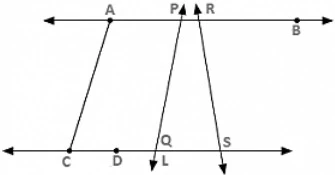Solution:

(i) Five line segments AB, CD, AC, PQ, and DS

(ii) Five rays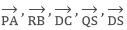(iii) Four collinear points. C, D, Q, S

(iv)Two pairs of non-intersecting line segments AB and CD, AB and LS.

## Topics & Subtopics Covered in NCERT Mathematics book for Class 9 Chapter 5 Introduction to Euclid’s Geometry

• With Euclid’s Geometry, the different aspects like a point, a line, and a plane, all are explained clearly.
• Postulates are also defined as the assumption, which is a universal truth.
• The theorems exhibit deductive reasoning.
• The different Euclid’s axioms and postulates, along with the different rules and laws associated with them.
• The fifth postulate of Euclid’s has been defined in much detail.
• The Euclid’s geometry helps in understanding many different geometrical concepts, which can be termed as non-Euclid’s geometry.

## Significance of NCERT Mathematics Book Class 9

• The NCERT maths book helps the students in learning about the different maths concepts and formula in depth and with better understanding.
• The NCERT maths book helps in strengthening the maths knowledge of the students.
• The NCERT book provides the students with multiple examples that help in understanding each chapter perfectly.
• At the end of the NCERT book, there is a different appendix, which is provided for the students to enhance their maths knowledge and skills.
• The NCERT book is perfect for preparing for different competitive examination. Maths is a crucial subject that is important for all the competitive examination. Thus, for all the students belonging to various fields can perfect their maths skills with the NCERT math book.
• The NCERT book offers the students with the facility to implement their hard efforts in a systematic manner, as each chapter has been systematically divided, to utilize the efforts and knowledge appropriately.

Students must start practicing for their exams with the use of NCERT books and clear their doubts, if any, by using Doubtnut website or app. It will help them save their precious time and it will also be more productive for them than previously.